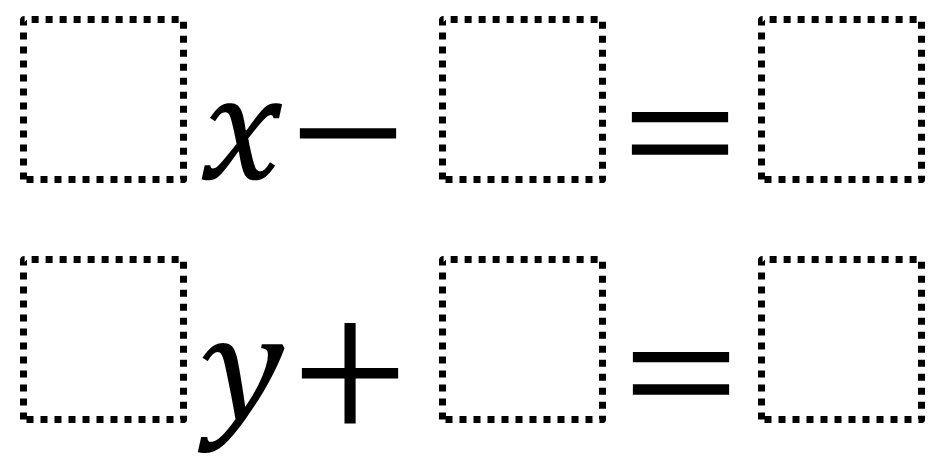# Two-Step Equations 3

Directions: Using the digits 1 to 9, at most one time each, fill in the boxes to find the largest (or smallest) possible values for the sum of x and y.### Hint

Where do you want to put the largest numbers? Where should you put the 1 and the 2?

The largest value is 19 which can be achieved by the following:
1x-8=9 and 2y+3=7

The smallest value is -6.125 which can be achieved by the following:
8x-3=4 and 1y+9=2

Source: Erick Lee

## Two Step Inequality with Fractional Coeffcient

Directions: Using the digits 1 to 7 at most one time each, place a digit …

1.Just a note that the second equation with the y value should have a subtraction sign vice an addition sign in order for the solution to be correct. (__y – __ = __)

•Sorry, I mean the first equation with the x. oops.

•Thanks for the heads up. I just fixed it.

2.I believe the answer should be as follows (this combines the results from TWO-STEP EQUATIONS 1 and TWO-STEP EQUATIONS 2):

The largest possible sum is 24, achieved with the following equations:
1x – 9 = 8 or 1x – 8 = 0 –> x = 17
1y – 2 = 9 –> y = 7

The least possible sum is -6 2/3, achieved with the following equations:
9x – 1 = 2 or 9x – 2 =1 –> x = 1/3
1y + 9 =2 –> y = -7

•Woops! I take that back. In the attempt that I cited as an answer, I ignored that I can’t repeat numbers that I’ve used already. That adds to the complexity!

My brain just grew. =)

3.I think finding the smallest positive value would add to this problem.

9x – 1 =2 and 8x +3 = 4 11/24 or .46

4.1x – 5 = 4
x = 9
2y + 2 = 8
9 + 3 = 15
I can only use numbers 1-9 once and i cant use negative numbers right( 1-9 it doesn’t say negative).

•Were allowed to use negatives for this equasion right?

5.7x – 6 = 8
x = 2

4y + 5 = 9
y = 1